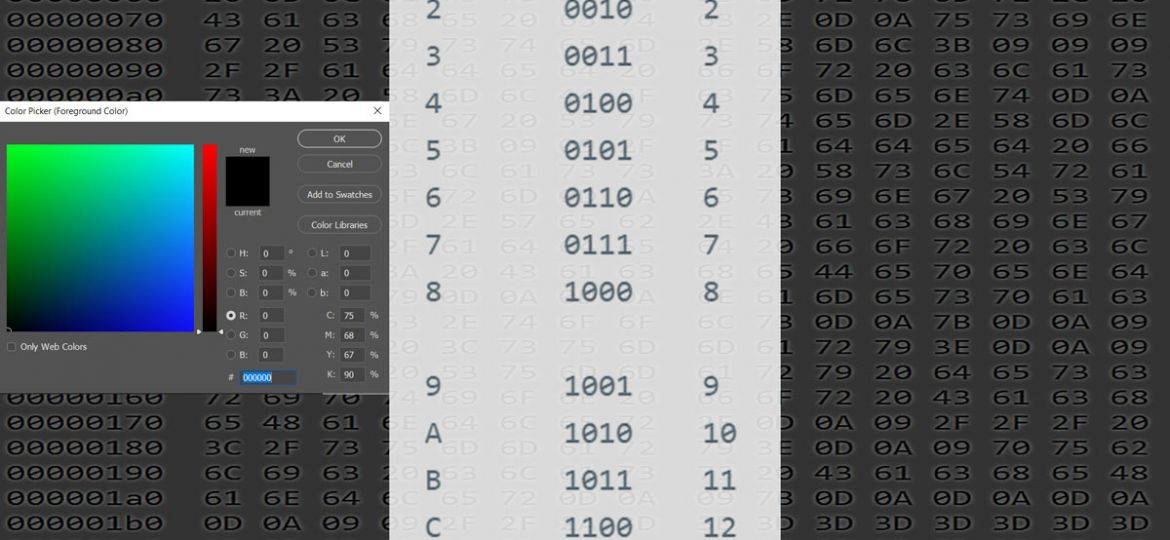# HEXADECIMAL System — what is it and how to use it in the real worldOnce upon a time it was a part of the basics a programmer needed to know, nowadays with frameworks that encapsulate all low level operations it is not as well known anymore. And professions like UI designers, photographers, video and photo editors can actually benfit from really understanding what HEX means instead of just using hexadecimal expressions.

Here we go, and I try to keep it as simple as possible.

### Where are hex values used?

For example in web design: colors in CSS are represented through 3 hex values (RGB), e.g. #c1c1c1 (a gray value), unless you use named colors. Or when it comes to the topic: encoding of special characters, e.g. German ‘Umlaute’, in XML or simply an editor. In order to better understand what really happens it is useful to understand the hexadecimal system.

Another example is Photo and Video editing which has multiple tasks that interface with hex values.

The decimal system is based on the value ’10’, the hexadecimal system is based on ’16’.

A brief recap: 1 byte is represented by 8 bits, meaning 4 bits for each half-byte (0000 0000).

A half-byte can reflect the decimal values 0 to 15; the hex system knows the values as 0 – 9 and A – F. Altogether 16 values.

See following table for the values that 4 bits can represent:

```hex	binary	dec
0	0000	0
1	0001 	1
2	0010 	2
3	0011 	3
4	0100 	4
5	0101 	5
6	0110 	6
7	0111 	7
8	1000 	8

9	1001 	9
A	1010 	10
B	1011 	11
C	1100 	12
D	1101 	13
E	1110 	14
F	1111 	15```

Again: altogether 16 different values.

‘F’ is the highest hex value 4 bits can represent, i.e. binary 1111 is in hex: F.

Therefore 2 hex values are needed to represent 1 byte, e.g. hex FF (== 8 bits: 1111 1111).

Different programming languages and environments have a different syntax and prefixes to indicate a hex value:

In XML, to represent Unicode chars, the prefix is: &#x (e.g. &#xAE is a ‘Registered Trademark sign’ and &#x263A is a Smiley). For a Registered Trademark sign in a XML file you would write either `&#xAE; (in hex) or &#174; (in decimal)`

In CSS, colors are prefixed with: # (e.g. #c1c1c1 is a gray) — hashtag hex-value is the common syntax to describe RGB colors.

Binary

Following you see the lowest and highest value 1 byte (8 bit) can have.

```| ............ 1 byte ...........|
|   half-byte    |   | half-byte |
----------------------------------
128 - 64 - 32 - 16   8 - 4 - 2 - 1	value represented by each bit (generic expr.: 2
n)
----------------------------------
0     0    0    0    0   0   0   0	results in: dec 0 (= hex '00')

1     1    1    1    1   1   1   1	results in: dec 255 (=hex 'FF')```
```Conversion from binary to decimal works by multiplying the bit value (0 or 1)
with the value
represented by the position of the bit (128+64+32+16+8+4+2+1 = 255)```

### Hex

In hex each position represents a half-byte, the highest value per byte is: hex FF (255).

Following some hex examples for 8bit (1-byte) and 16bit (2-byte) values: xFF, xAE, x111A and how to convert a hex value to a decimal value:

```|  byte  |   |byte|
4096 - 256 - 16 - 1 value represented by each position (generic expr.: 16n)
-------------------
F    F   results in decimal 255 ((1 x 15)=15 plus (16 x 15)=240)
A    E   results in decimal 174
1    1   1    A   results in decimal 4378 (1 x 4096 plus 1 x 256 plus 1 x 16 plus 10 x 1```

#### Following the 16n values for 1bye, 2 bytes, 3 bytes, 4 bytes

You can do the math by following above samples. Or just type the hex values into e.g. your Windows Calculator which converts in all directions very nicely.

#### Max. values for 16bit or 32bit

In the programmer’s world 16bit code, then 32bit code and now 64bit code is quite normal.
Basically the compiler does everything but the programmer must understand the maximum address  space which can be reached.

8bit (1 byte) = hex FF = decimal 255 (a range from 0 to 255, altogether 256 different values possible)

16bit (2 bytes) = hex FF FF = decimal 65,535 (that’s why you may encounter the term 64k as a limit so often, especially if the variable which holds the value is a 16 bit unsigned integer.
A signed integer reserves one of the bits to store the sign (+/-) and therefore the value range is only from −32,768 to +32,767 (signed).

32bit (4 bytes) = hex FF FF FF FF = decimal 4,294,967,295 (~ 4Gig). That’s why a 32-bit OS can only address 4GB Memory in your 32bit machine, even if you installed 8GB. That’s why you need a 64-bit system / OS.